# Tamil Nadu Board Samacheer Kalvi solutions for Class 4th Mathematics Term 1 Answers Guide chapter 2 - Numbers [Latest edition]

#### Chapters## Chapter 2: Numbers

Exercise 2.1Exercise 2.2Exercise 2.3Exercise 2.4Exercise 2.5Exercise 2.6Exercise 2.7Exercise 2.8Exercise 2.3c
Exercise 2.1 [Pages 20 - 21]

### Tamil Nadu Board Samacheer Kalvi solutions for Class 4th Mathematics Term 1 Answers Guide Chapter 2 NumbersExercise 2.1 [Pages 20 - 21]

Exercise 2.1 | Q A. (i) | Page 20

Write the following number in words

1006 - ____________________________

Exercise 2.1 | Q A. (ii) | Page 20

Write the following number in words

6327 - _________________

Exercise 2.1 | Q A. (iii) | Page 20

Write the following number in words

9097 - __________________________

Exercise 2.1 | Q A. (iv) | Page 20

Write the following number in words

10,000 - _________________________

Exercise 2.1 | Q A. (v) | Page 20

Write the following number in words

8906 - ____________________________

Exercise 2.1 | Q B. (i) | Page 21

Write the numeral for the following.

Seven thousand and sixty four - __________

Exercise 2.1 | Q B. (ii) | Page 21

Write the numeral for the following.

Nine thousand three hundred and forty - __________

Exercise 2.1 | Q B. (iii) | Page 21

Write the numeral for the following.

Five thousand six hundred and seventy three - _________

Exercise 2.1 | Q B. (iv) | Page 21

Write the numeral for the following.

Ten thousand - __________

Exercise 2.1 | Q B. (v) | Page 21

Write the numeral for the following.

Four thousand three hundred and six - ___________

Exercise 2.1 | Q C. (i) | Page 21

Ramu went to a bank to deposit Rs. 7500. In the deposit form, he has to fill up the amount in words. Could you please help him?

Exercise 2.1 | Q C. (ii) | Page 21

Find the sum of the greatest two digit and the greatest three digit numbers. Write the number names of that sum

Exercise 2.2 [Pages 22 - 23]

### Tamil Nadu Board Samacheer Kalvi solutions for Class 4th Mathematics Term 1 Answers Guide Chapter 2 NumbersExercise 2.2 [Pages 22 - 23]

Exercise 2.2 | Q 1 | Page 22

Circle the odd number in the following.

9001, 8002, 7603, 6542, 4875, 3882, 3217

Exercise 2.2 | Q 2 | Page 22

Circle the even number in the following.

6231, 5920, 4812, 2121, 1234, 9528, 3946

Exercise 2.2 | Q 3 | Page 23

Choose the even number from the following and write the number and number name

6501, 4706, 3999, 4001, 3848

 Number Number name
Exercise 2.2 | Q 4 | Page 23

Pick out the even number and write their name in the table given below.

4703, 3206, 2003, 4017, 2001

 Number Number Name
Exercise 2.3 [Pages 24 - 25]

### Tamil Nadu Board Samacheer Kalvi solutions for Class 4th Mathematics Term 1 Answers Guide Chapter 2 NumbersExercise 2.3 [Pages 24 - 25]

Exercise 2.3 | Q 1. a) | Page 24

Find the face value and place value of the digits coloured in the given numbers.

1379

Exercise 2.3 | Q 1. b) | Page 24

Find the face value and place value of the digits coloured in the given numbers.

9876

Exercise 2.3 | Q 1. c) | Page 24

Find the face value and place value of the digits coloured in the given numbers.

5136

Exercise 2.3 | Q 1. d) | Page 24

Find the face value and place value of the digits coloured in the given numbers.

8965

Exercise 2.3 | Q 1. e) | Page 24

Find the face value and place value of the digits coloured in the given numbers.

2010

Exercise 2.3 | Q 1. f) | Page 24

Find the face value and place value of the digits coloured in the given numbers.

4038

Exercise 2.3 | Q 2 | Page 25

Complete the given table.

 Number Expanded Form a 6785 b 4000 + 200 + 90 + 6 c 3000 + 300 + 20 + 7 d 9999 e 5000 + 70 + 1 f 2934
Exercise 2.3 | Q 3 | Page 25

Circle the correct one.

 a 5 thousand + 3 hundred + 2 ones 5320, 5302 b The place value of 5 in 3758 50, 500 c Three thousand six hundred and sixty 3060, 3660 d 4000 + 600 + 90 4690, 4609
Exercise 2.4 [Page 27]

### Tamil Nadu Board Samacheer Kalvi solutions for Class 4th Mathematics Term 1 Answers Guide Chapter 2 NumbersExercise 2.4 [Page 27]

Exercise 2.4 | Q 1. a) | Page 27

Write the following number in ascending order.

7631, 9987, 7634, 5436, 8918

Exercise 2.4 | Q 1. b) | Page 27

Write the following numbers in ascending order.

4096, 3096, 3099, 2473, 3172

Exercise 2.4 | Q 1. c) | Page 27

Write the following numbers in ascending order.

5201, 5627, 4325, 9999, 9801

Exercise 2.4 | Q 2. a) | Page 27

Write the following numbers in descending order.

3435, 3670, 139, 3267, 6544

Exercise 2.4 | Q 2. b) | Page 27

Write the following numbers in descending order.

2785, 3605, 2782, 236, 9801

Exercise 2.4 | Q 2. c) | Page 27

Write the following numbers in descending order.

6998, 6987, 6898, 7801, 8979

Exercise 2.4 | Q 3 | Page 27

Arrange the milestones given below in both the orders.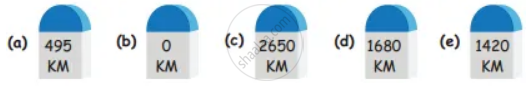Exercise 2.5 [Pages 29 - 30]

### Tamil Nadu Board Samacheer Kalvi solutions for Class 4th Mathematics Term 1 Answers Guide Chapter 2 NumbersExercise 2.5 [Pages 29 - 30]

Exercise 2.5 | Q 1 | Page 29

Form the greatest and smallest number using the given digits only once.

 Digits Greatest number Smallest number a 1, 4, 3, 7 b 5, 0, 9, 3 c 6, 7, 1, 5 d 3, 2, 0, 9 e 7, 3, 2, 8 f 4, 6, 0, 2 g 9, 1, 4, 0
Exercise 2.5 | Q 2. a) | Page 30

Circle ○ the smallest number and tick (✓) the greatest number:

2715, 2175, 2517, 2157, 2275

Exercise 2.5 | Q 2. b) | Page 30

Circle ○ the smallest number and tick (✓) the greatest number.

6238, 2386, 3862, 8623, 9378

Exercise 2.5 | Q 2. c) | Page 30

Circle ○ the smallest number and tick (✓) the greatest number.

9345, 9646, 3408, 1425, 2000

Exercise 2.5 | Q 2. d) | Page 30

Circle ○ the smallest number and tick (✓) the greatest number.

5931, 1370, 4000, 2000, 3000

Exercise 2.5 | Q 2. e) | Page 30

Circle ○ the smallest number and tick (✓) the greatest number.

6000, 7000, 5000, 4000, 9000

Exercise 2.5 | Q 2. f) | Page 30

Circle ○ the smallest number and tick (✓) the greatest number.

2468, 4279, 5090, 7906, 6270

Exercise 2.5 | Q 2. g) | Page 30

Circle ○ the smallest number and tick (✓) the greatest number.

7692, 8296, 3241, 9276, 4291

Exercise 2.6 [Pages 31 - 33]

### Tamil Nadu Board Samacheer Kalvi solutions for Class 4th Mathematics Term 1 Answers Guide Chapter 2 NumbersExercise 2.6 [Pages 31 - 33]

Exercise 2.6 | Q 1. i | Page 31

Fill in the boxes.

4634 + square = 4634

Exercise 2.6 | Q 1. ii | Page 31

Fill in the boxes.

2134 + 1 = square

Exercise 2.6 | Q 1. iii | Page 31

Fill in the boxes.

5349 + 0 = square

Exercise 2.6 | Q 1. iv | Page 31

Fill in the boxes.

1435 + 1923 = 1923 + square

Exercise 2.6 | Q 1. v | Page 31

Fill in the boxes.

3457 + square = 3458

Exercise 2.6 | Q 2. (i) | Page 31

Th H  T O
3  2  5  4
+ 1  4  2  4
_________

Exercise 2.6 | Q 2. (ii) | Page 31

Th H  T O
2  1  3  5
+ 3  3  4  2
_________

Exercise 2.6 | Q 2. (iii) | Page 31

Th H  T O
3  7  6  2
+ 3  1  3  7
__________

Exercise 2.6 | Q 2. (iv) | Page 31

Th H  T O
1  4  3  3
+  4  5  5  2
__________

Exercise 2.6 | Q 3 | Page 31

Add: 2713 + 104 + 1172 + 6010

Exercise 2.6 | Q 4 | Page 31

A man visited a furniture shop. He bought a bed for ₹ 2100, a dining table for ₹ 3500, and six chairs for ₹ 4200. How much money did he pay to the shopkeeper?

Exercise 2.6 | Q 5. (a) | Page 31

Create word problem for the addition facts given below.

3094 + 7923 = 11,017

Exercise 2.6 | Q 5. (b) | Page 31

Create word problem for the addition facts given below.

8309 = 2309 + square

Exercise 2.6 | Q 6. (a) | Page 32

Create addition stories using the picture and number given below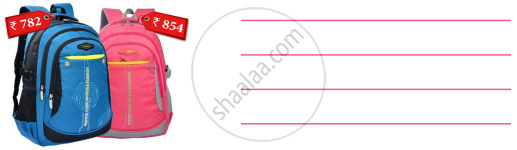Exercise 2.6 | Q 6. (b) | Page 32
 Child birth between 2017 and 2018 are given below District Children Trichy Urban 1032 Rural 2030 Ariyalur Urban 1205 Rural 4097 Kanchipuram Urban 2104 Rural 4034 Chennai Urban 1430 Rural 1023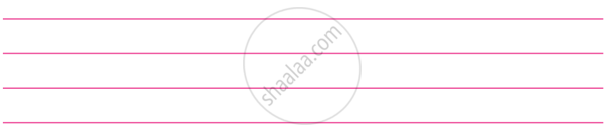Exercise 2.6 | Q 7 | Page 32

Fill in the circles using 1400, 1500, 1600, 1700, 1800 and 1900 so that the three numbers along each line add up to 5000.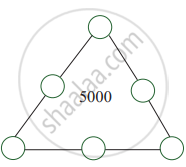Exercise 2.6 | Q 8 | Page 33

Fill in the box with appropriate number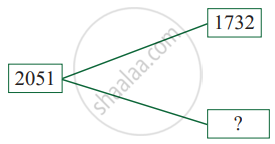Exercise 2.7 [Pages 34 - 35]

### Tamil Nadu Board Samacheer Kalvi solutions for Class 4th Mathematics Term 1 Answers Guide Chapter 2 NumbersExercise 2.7 [Pages 34 - 35]

Exercise 2.7 | Q 1. a) | Page 34

216, 3422, 4019, 497

Exercise 2.7 | Q 1. b) | Page 34

1002, 2347, 1976, 2005, 2007

Exercise 2.7 | Q 1. c) | Page 34

1978, 1965, 2704, 473

Exercise 2.7 | Q 2 | Page 34

Add the total amount of the following 4 piggy banks.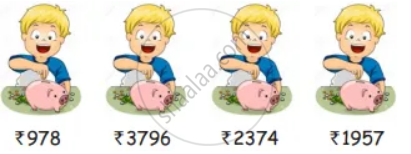Exercise 2.7 | Q 3 | Page 34

The sum of 1215 + 2367 + 1673 + 3120 = __________

• 8585

• 8225

• 8375

• 8285

Exercise 2.7 | Q 4 | Page 34

2076 + 276 + 2974 + 1751 =

• 9561

• 7077

• 7377

• none of these

Exercise 2.7 | Q 5 | Page 34

What is the sum of five hundred and fifteen tens?

• 650

• 550

• 5150

• 6150

Exercise 2.7 | Q 6 | Page 34

The sum of the greatest 3 digit number and the smallest 4 digit number is

• 1999

• 1099

• 1990

• 9999

Exercise 2.7 | Q 7 | Page 34

9999 + 1 = ___________

• 10,000

• 1000

• 1001

• 10001

Exercise 2.7 | Q 8 | Page 35

In a village the number of males is 4154 and the number of females is 4221. Find the total population in the village?

Exercise 2.7 | Q 9 | Page 35

A refrigerator cost ₹ 6543 and a DVD player cost ₹ 3412. What is the total cost?

Exercise 2.8 [Page 35]

### Tamil Nadu Board Samacheer Kalvi solutions for Class 4th Mathematics Term 1 Answers Guide Chapter 2 NumbersExercise 2.8 [Page 35]

Exercise 2.8 | Q 1 | Page 35

9  7  6  4
3  4  2  3

Exercise 2.8 | Q 2 | Page 35

7  9  8  6
4  5  2  4

Exercise 2.8 | Q 3 | Page 35

4  7  8  5
2  4  6  2

Exercise 2.3c [Pages 37 - 38]

### Tamil Nadu Board Samacheer Kalvi solutions for Class 4th Mathematics Term 1 Answers Guide Chapter 2 NumbersExercise 2.3c [Pages 37 - 38]

Exercise 2.3c | Q A. 1. | Page 37

Subtract

TH  H  T  O
3   4   4  5
−   1   3   4  8

Exercise 2.3c | Q A. 2. | Page 37

Subtract

TH  H  T  O
4   9   6  5
−   2   4   4  6

Exercise 2.3c | Q A. 3. | Page 37

Subtract

TH  H  T  O
6   5   7  0
−   3   3   9  7

Exercise 2.3c | Q A. 4. | Page 37

Subtract

TH  H  T  O
8   9   5   3
−   5   9   6  4

Exercise 2.3c | Q B. a) | Page 37

Find the difference between given numbers.

4352 and 5020

Exercise 2.3c | Q B. b) | Page 37

Find the difference between given numbers.

1438 and 3370

Exercise 2.3c | Q B. c) | Page 37

Find the difference between given numbers.

2526 and 8431

Exercise 2.3c | Q B. d) | Page 37

Find the difference between given numbers.

3361 and 9000

Exercise 2.3c | Q C. 1. | Page 37

The sum of two numbers is 7036, one number is 3168. Find the other number

Exercise 2.3c | Q C. 2. | Page 37

A man had Rs 9200 in the bank. He withdrew Rs 2756. How much money does he have in the bank now?

Exercise 2.3c | Q D. a) | Page 37

Create the subtraction story problems for the details given below.

1997 – 1968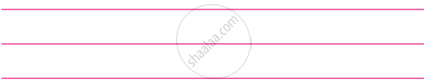Exercise 2.3c | Q D. b) | Page 37

Create the subtraction story problems for the details given below.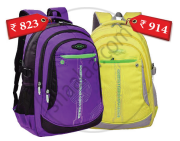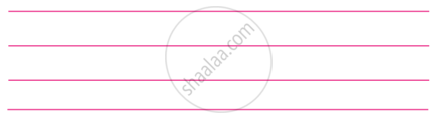Exercise 2.3c | Q D. c) | Page 38

Create the subtraction story problems for the details given below.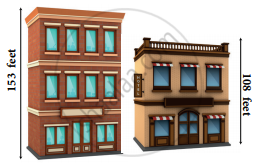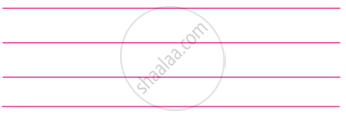## Chapter 2: Numbers

Exercise 2.1Exercise 2.2Exercise 2.3Exercise 2.4Exercise 2.5Exercise 2.6Exercise 2.7Exercise 2.8Exercise 2.3c## Tamil Nadu Board Samacheer Kalvi solutions for Class 4th Mathematics Term 1 Answers Guide chapter 2 - Numbers

Tamil Nadu Board Samacheer Kalvi solutions for Class 4th Mathematics Term 1 Answers Guide chapter 2 (Numbers) include all questions with solution and detail explanation. This will clear students doubts about any question and improve application skills while preparing for board exams. The detailed, step-by-step solutions will help you understand the concepts better and clear your confusions, if any. Shaalaa.com has the Tamil Nadu Board of Secondary Education Class 4th Mathematics Term 1 Answers Guide solutions in a manner that help students grasp basic concepts better and faster.

Further, we at Shaalaa.com provide such solutions so that students can prepare for written exams. Tamil Nadu Board Samacheer Kalvi textbook solutions can be a core help for self-study and acts as a perfect self-help guidance for students.

Concepts covered in Class 4th Mathematics Term 1 Answers Guide chapter 2 Numbers are Number Sequence Upto 10,000, Concept of Even and Odd Number, Expansion Form of Numbers, Compare Numbers in Ascending and Descending Order, Compare Number by Forming Numbers from a Given Digits, Addition and Subtraction.

Using Tamil Nadu Board Samacheer Kalvi Class 4th solutions Numbers exercise by students are an easy way to prepare for the exams, as they involve solutions arranged chapter-wise also page wise. The questions involved in Tamil Nadu Board Samacheer Kalvi Solutions are important questions that can be asked in the final exam. Maximum students of Tamil Nadu Board of Secondary Education Class 4th prefer Tamil Nadu Board Samacheer Kalvi Textbook Solutions to score more in exam.

Get the free view of chapter 2 Numbers Class 4th extra questions for Class 4th Mathematics Term 1 Answers Guide and can use Shaalaa.com to keep it handy for your exam preparation